# 2.0 SGC Tri-Coordinates

The current system used by astronomers to locate a star is based on Earth polar sky positions; Right Ascension and declination; and an estimated distance. These measurements could be transferred to a Earth polar Tri-Coordinate system using trigonometry. It is, however, more preferable to have a 3D stellar map based on galactic polar and not Earth polar. This means the Earth polar based measurements should first be transferred to galactic polar. Once such galactic polar measurements are known, the SGC Tri-Coordinate's X,Y and Z may then be found.The following table, and other tables like this one throughout the paper, can be used as a guideline to what formulae are being discussed in the following text. Such tables do not always contain formulae, but help to sort out what is needed to find what. This table shows what known data is required to find the new galactic based data. The 'Data In' column holds already known values and the 'Data Out' column shows newly found data based on galactic polar.

`                                                      2.0.1`

### SGC Tri-Coordinates

Source Data In Data Out
known  Apparent Stellar Distance to the star (ASD
known  Earth Polar Right Ascension of the star (RA Galactic Right Ascension (Gr
known  Earth Polar declination of the star (d Galactic declination (Gd
known  Earth polar declination of the galactic north (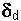known  Earth polar Right Ascension of the North Galactic Pole. (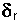)
Earth polar angle to the galactic center (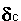• Drop Point of Star to XY plane. (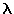• Distance from 0,0,0 coordinates

• to () Drop Point of Star (DDP).
• Apparent SGC values for X, Y, Z and T.
• ### 2.1 The X, Y, Z coordinates defined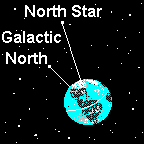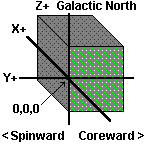The X. Y and Z coordinates are measured in light years. The direction of the three axis are arbitrary. The axis for the SGC system is set as follows:

• The X axis runs positive in the direction of movement of the sun rotating around the galaxy. (Direction of the Cygnus constellation).

•

• The Y axis runs negative or coreward to the galactic center (between the constellation Sagittarius and Scorpio). The Y axis runs positive or rimward to the galactic rim. (Direction of Taurus near the Pleiades and Hyades clusters).

•

• The Z axis runs positive through galactic north in the constellation Coma Berenices and negative through galactic south near to Tau Ceti.

### 2.2 Formulae to find the SGC Tri-Coordinates

The transformation of Right Ascension and declination values from Earth polar to galactic polar uses established formulae. To find the galactic declination of a star the formula is:

`                                                      2.2.1`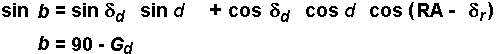Where angle b is a variable and may be positive or negative, Gd = galactic declination of the star (from 0° to 180°),= the Earth polar declination of the north galactic pole, RA = Earth polar Right Ascension of the star, d = Earth polar declination of the star, and= Earth polar Right Ascension of the north galactic pole.

To determine galactic Right Ascension either of the following applies:

`                                                      2.2.2`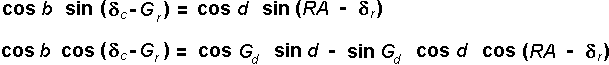Where= the angle from Earth polar to the galactic center. (In the SGC system, the direction to the galactic center would be Y negative.) Gr = The galactic Right Ascension of the star.

### 2.3 Transfer of galactic based values to SGC coordinates

One difficulty in resolving numeric values for X and Y is that the galactic Right Ascension (Gr) must be first transposed from hours and minutes to values in degrees and then the X and Y new galactic values be assigned the arbitrary positive or negative values, depending in which sector the star is found.

The SGC system creates four possible quadrants of X and Y. Using the angle to galactic center, Y negative, as the 0° mark and moving in a counter clockwise direction as seen from above, matching the Right Ascension. The following applies:

`                                                      2.3.1`Further division can be made of these four quadrants into eight sectors where four sectors have positive Z values above or north of the line from our Sun to the galactic center and four sectors below or south of this line. I have arbitrarily named the galactic sectors using the Greek alphabet; alpha, beta, gamma, delta, kappa, sigma, tau and omega.

Where the sectors are named from above in a counter-clockwise rotation:

`                                                     2.3.2`
The Galactic Sectors
Alpha
X+, Y+, Z+
Beta
X+, Y-, Z+
Gamma
X-, Y+, Z+
Delta
X-, Y-, Z+

#### - - - The Galactic Equator- - -

Kappa
X+, Y+, Z-
Sigma
X+, Y-, Z-
Omega
X-, Y+, Z-
Tau
X-, Y-, Z-
Illustration 2.3.3 shows a sample of these SGC sectors.

`                                                     Illustration 2.3.3`
Updated version coming

Transferring galactic Right Ascension, galactic declination, and distance to the star to X, Y, and Z coordinates becomes an exercise in trigonometry.

`                                                     Graph 2.3.4`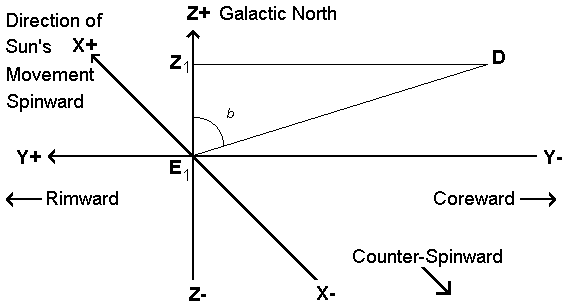Graph 2.3.4 shows a Z coordinate value for a star at D. The X, Y, and Z axis are set, as previously stated, to the SGC system; where X + = Spinward, the direction of the Sun's galactic orbit, X - = Counter-Spinward, Y + = Rimward, towards the outer rim of our galaxy, Y - = Coreward, to the center of the galaxy, Z + equals galactic North Pole and finally, Z - = the galactic South Pole. Angle b shows the galactic declination of star D. E1Z1 = the Z coordinate value of star at D.

It can be seen that if angle b and the distance from E1D is known, it becomes a simple matter to find E1Z1. This value is the the star D' SGC Z coordinate value.

`                                                      2.3.5`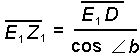One more value is needed to find before resolving a three coordinate solution. The value needed is the distance from the 0,0,0 coordinate to the drop point of the star to the XY plane. This distance is designated as Distance to Drop Point (DDP). This distance is obviously smaller than the Apparent Stellar Distance (ASD) because the ASD is the hypotenuse and the DDP is an adjacent side.

`                                                      Graph 2.3.6`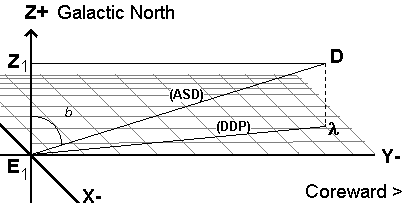(The galactic center is considered the starting point of the galactic Right Ascension and thereby makes any X values positive when the galactic Right Ascension is between 0° and 180°.The X values are negative when the galactic Right Ascension is between 180 degrees and 360 degrees.)
The formula to find DDP is:

`                                                      2.3.7`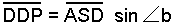Therefore to transfer galactic based values of Right Ascension and declination to the SGC X coordinate:

`                                                      2.3.8`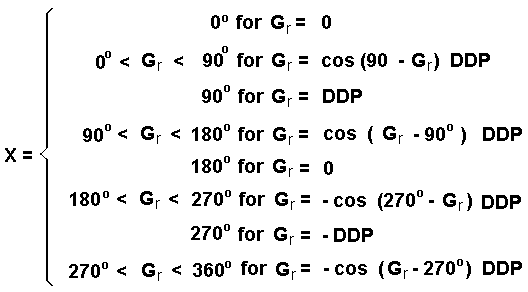Transfer to the SGC - Y coordinate can be derived in a similar fashion:

`                                                      2.3.9`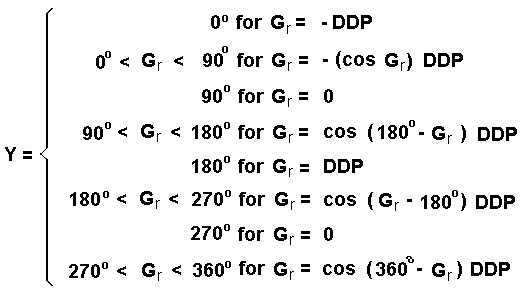To transfer galactic based values to the SGC - Z coordinate, you must use the Apparent Steller Distance (ASD) and not the DDP:

`                                                      2.3.10`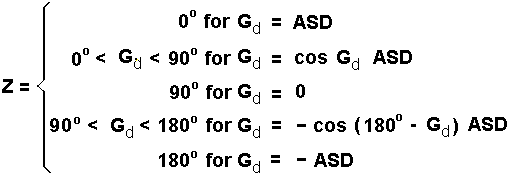It must be remembered that galactic declination may take the values from 0° to 180° and galactic Right Ascension may take values from 0° to less than 360°.

This defines the trigometric solution to finding the X, Y and Z coordinates based on the galactic Right Ascension, galactic declination and Apparent Stellar Distance.

#### Forward to Chapter 3 - The Time Coordinate

Backward to Chapter 1 - Principal of the SGC System